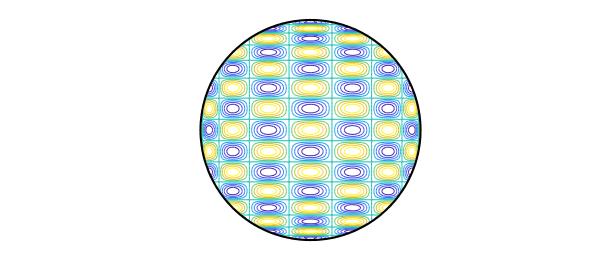### 1. Numerical integration over the disk

In studying cubature formulas, we needed to compute the integrals of products of Chebyshev polynomials $T_j(x) T_k(y)$ over the unit disk, like this one:

T8 = chebpoly(8); T16 = chebpoly(16);
s = linspace(-1,1,160); [xx,yy] = meshgrid(s,s);
ff = T8(xx).*T16(yy);
ff(xx.^2+yy.^2>1) = NaN;
contour(s,s,ff), axis equal off
hold on, plot(chebfun('exp(1i*x)',[0 2*pi]),'k'), hold offIf $j$ or $k$ is odd, the integral is zero, but even if they are both even, to our surprise, we found that the integrals are still usually zero! In fact, a nonzero result only shows up if $j$ and $k$ differ by 0 or 2. Thus the function plotted above, for example, has integral exactly zero over the disk. This is not obvious.

Speaking in general, suppose we want to integrate a smooth function $f(r,t)$ numerically over the unit disk, where $r$ is radius and $t$ is angle. One could use Diskfun, but here, we use standard Chebfun. Let's assume that $f$ is defined by a function that accepts a scalar $r$ and a vector $t$, like this one:

f = @(r,t) 1 + 0*t;

Here is a numerical confirmation that the integral of $1$ is $\pi$:

format long
fr = @(r) r*sum(chebfun(@(t) f(r,t),[0,2*pi],'trig'));
I = sum(chebfun(@(r) fr(r),[0 1]))
Iexact = pi
I =
3.141592653589793
Iexact =
3.141592653589793


For the function $f(r,t) = r^2$, the integral is $\pi/2$:

f = @(r,t) r^2 + 0*t;
fr = @(r) r*sum(chebfun(@(t) f(r,t),[0,2*pi],'trig'));
I = sum(chebfun(@(r) fr(r),[0 1]))
Iexact = pi/2
I =
1.570796326794897
Iexact =
1.570796326794897


For the function $f(r,t) = r^2 \cos^2(t)$, the integral is $\pi/2$:

f = @(r,t) r^2*cos(t).^2;
fr = @(r) r*sum(chebfun(@(t) f(r,t),[0,2*pi],'trig'));
I = sum(chebfun(@(r) fr(r),[0 1]))
Iexact = pi/4
I =
0.785398163397448
Iexact =
0.785398163397448


### 2. Numerical integration of products of Chebyshev polynomials

What about those products of Chebyshev polynomials? Here is a matrix showing the numerically computed integrals for $k = 0,2,4,6,8,10$. As claimed above, the matrix is tridiagonal.

tic
I = zeros(6);
format short
for j = 0:2:10
Tj = chebpoly(j);
for k = 0:2:j
Tk = chebpoly(k);
f = @(r,t) Tk(r*cos(t)).*Tj(r*sin(t));
fr = @(r) r*sum(chebfun(@(t) f(r,t),[0,2*pi],'trig'));
I(1+j/2,1+k/2) = sum(chebfun(@(r) fr(r),[0 1]));
end
end
I = I + tril(I,-1)'
time_elapsed_in_seconds = toc
I =
3.1416   -1.5708   -0.0000    0.0000   -0.0000    0.0000
-1.5708    0.5236    0.2618    0.0000   -0.0000   -0.0000
-0.0000    0.2618   -0.1047   -0.1571    0.0000   -0.0000
0.0000    0.0000   -0.1571    0.0449    0.1122    0.0000
-0.0000   -0.0000    0.0000    0.1122   -0.0249   -0.0873
0.0000   -0.0000   -0.0000    0.0000   -0.0873    0.0159
time_elapsed_in_seconds =
3.7174


### 3. Analytic expressions for the integrals

Let $I_{jk}$ denote the integral of $T_j(x) T_k(y)$ over the unit disk. The following explicit formulas derived by the first author (details not reported here) give the integrals:

$$I_{00} = \pi,$$

$$I_{02} = I_{20} = -{\pi\over 2},$$

$$I_{kk} = {\pi (-1)^{k/2} \over 2 - 2k^2} \quad \hbox{(k even, k\ge 2)},$$

$$I_{k,k+2} = I_{k+2,k} = {\pi (-1)^{1+k/2} \over 4k + 4} \quad \hbox{(k even, k\ge 2)},$$

In all other cases $I_{jk} = 0$.

Using these formulas, we can reproduce the matrix above as follows:

I = zeros(6);
I(1,1) = pi;
I(2,1) = -pi/2;
for k = 2:2:10
I(1+k/2,1+k/2) = pi*(-1)^(k/2)/(2-2*k^2);
end
for k = 2:2:8
I(2+k/2,1+k/2) = -pi*(-1)^(k/2)/(4*k+4);
end
I = I + tril(I,-1)'
I =
3.1416   -1.5708         0         0         0         0
-1.5708    0.5236    0.2618         0         0         0
0    0.2618   -0.1047   -0.1571         0         0
0         0   -0.1571    0.0449    0.1122         0
0         0         0    0.1122   -0.0249   -0.0873
0         0         0         0   -0.0873    0.0159


### 4. Application to integration of general functions

The results of the last section imply that there is an immediate way to compute the integral of a chebfun2 over a disk: just take the appropriate linear combination of its bivariate Chebyshev coefficients. After this example was initially written, we developed this idea into a Chebfun2 sumdisk command. See the example "Sumdisk for integration over a disk".